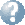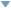Online Reference Dictionary, Encyclopedia & moreby: WordStarts with Ends withMentionsteaching:topics:calculus:notation

## Three notations for Calculus

### Why three?

The three most commonly used come from different perspectives. Newton was developing what is now called classical dynamics, and concerned principally with forces and the movement of objects — considered as changes over time. The big idea behind calculus concerning infinitessimals and infinite sums was new, and very contested, so he began his book with a traditional geometric analysis of planetary motions. His notation avoids making the infinitessimals or calculus explicit, refering instead to the physics application of them. Exploring this physics was the original motivation for developing the mathematics.

Leibniz was primarily concerned with that mathematics, which is much more generally applicable than just physics, and not necessarily about time. This notation explicitly refers to the underlying mathematical ideas, a concept quite distinct from any particilar application. It is a mathematical language, dealing with generalised number, ratios and sums — number which might be applied to specific measurements while modelling in one or another field. It is a much more flexible notation, much more extensible.

Lagrange was a century later, a mathematician. He worked on the three body problem in dynamics (in particular the Sun - Earth - Moon behavior), and a great deal more. He created a new mathematical formulation of dynamics that focussed on functions, and functions of functions. His notation reflects this, it explicitly references functions and the mapping of functions to new functions, rather than the ratios or sums of the changing values of these. An analogy here could be the difference between procedural and functional computer programming paradigms.

### some context ...

Newton’s was often used as more succinct and descriptive of the immediate application. The idea embodied in that notation — physical systems described by Cartesian coordinates in a euclidean space evolving over a completely independent time in ways calculatable using the masses and the changing forces involved — has been inappropriate in most physics developed since 1905. This was called Dynamics. It was the fundamental insight of their time. It had taken several generations and well over a century of pretty solid work to get there. It remains a very good approximation for most practical purposes. Certain details of quantum mechanics still depend on that spacial-temporal assumption (where efforts to formulate the equations in relativistic terms have failed, and the small scale and times involved mean this is not numerically significant).

It is interesting to note that exact, closed form, solutions to most dynamic problems involving feedback (such as forces between objects that determine their movement which in turn changes those forces) are not possible, and that even numerical solutions (evolving a system step by step) are very limited. ‘Chaos’ is the mathematics that deals with this idea, showing that the evolution of even quite simple systems over time is overwhelmingly dependent on very small variations of the initial conditions. There is no exact, general solution to the three body problem — it is not possible to make equations giving the position of three stars orbiting each other explicitly as a function of time (unless we are looking at some special cases). This is reflected in the stars we observe. Binary stars are very common, trinaries consisting of a close binary and another much more distant star are found, more complex pairings certainly exist, planets and moons around a star are stable when they are, effectively, independent binary systems. The three- or four-star systems we know that are not pairs of pairs are in areas of intense star formation, and presumably will split up, perhaps launching one as another of the high-velocity stars that move rapidly through, and out of, galaxies.

There are other very important physical models that are based on describing the whole system over a period of time, as opposed to a snapshot in time or their continuous or stepwise evolution through time determined by dynamically changing forces, acceleration, momentum and such. They existed in some form in classical Greek times. They can seem rather challenging in terms of our usual ideas of causality yet nevertheless are very useful and important. The cutting edge of physics today includes ideas that might radically alter our sense of time and space, at least at the fundamental, philosophical level. But that is for later — just to get started requires a very deeply mathematical view and a strong sense of the physics up to now — at school we have barely started that journey in either field.

### Newton

… refers directly to rates of change over time, which is the original motivation for calculus. We can explore these rates of change numerically (like Feynman did when introducing Newton’s Dynamics), geometrically (like Newton did with Kepler’s planetary laws when introducing Dynamics originally) or algebraically — using calculus, finding potentially stable systems (which are specific closed form solutions to a set of differential equations describing the feedbacks involved) then looking for examples of these experimentally — which was the focus a lot of 20th century particle physics.

His is the classical mechanics perspective, and a very good place to start.

Taking x to represent position, v velocity and $\boldsymbol{v}$ the velocity vector then …
\begin{align*} \dot x &&& \text{is the rate of change of position (that is velocity),} \\ \ddot x &&& \text{is the rate of change of the rate of change of position (so acceleration),} \\ \dot v &&& \text{is the rate of change of velocity (and so also acceleration) and} \\ \dot{\boldsymbol{v}} &&& \text{is the same but as a vector, so no longer in just one dimension.} \end{align*}

It is useful to use each of these symbols when talking about such rates of change over time, even before we consider the calculus methods of modelling and working with these values.

### Leibniz

… is more explicit about how this relates to equations and graphs, it is much more extendable to many of the other fields where calculus is used. It is a more mathematical perspective. It was developed simultaneously with Newton’s notation.

\begin{align*} \frac{{\rm d}y}{{\rm d}x} &&& \text{is the ratio of the instantaneous changes in two related values} \\ \frac{\rm d}{{\rm d}t}(4t^2+3) &&& \text{is between the values of the expression and the variable} \\ \text{repeated:}\quad\frac{{\rm d}^2}{{\rm d}x^2}(3x^3+5) &&& \text{means:}\quad\frac{\rm d}{{\rm d}x}\left(\frac{{\rm d}}{{\rm d}x}(3x^3+5)\right) \end{align*} The kind of calculus that we deal with at school treats each of these as a single symbol, but it does make sense to break them down into their parts, considering say $\ {\rm d}x\$ on its own. Doing so requires some care and a deep understanding of what is being done, but is a very powerful mathematical technique.

### Lagrange

… was a century later, and considers functions as entities which can be operated on. He used the function notation introduced earlier in the 18th century by Euler and others. This is another distinct mathematical perspective, and a language that facilitates a very different kind of thinking.

\begin{align*} {\rm f}(t) &&& \text{a function with values that depend on the variable } t \\ {\rm f}'(t) &&& \text{the function that is the derivative of that function} \\ {\rm f}^{\prime\prime}(t) &&& \text{the function that is the derivative of that derivative function} \\ \text{and even} \quad {\rm f}^{(n)}(t) &&& \text{the function that is the }n \text{th derivative of }{\rm f}(t) \end{align*}

teaching/topics/calculus/notation.txt · Last modified: 2021/01/11 14:08 by simon

### Page Tools